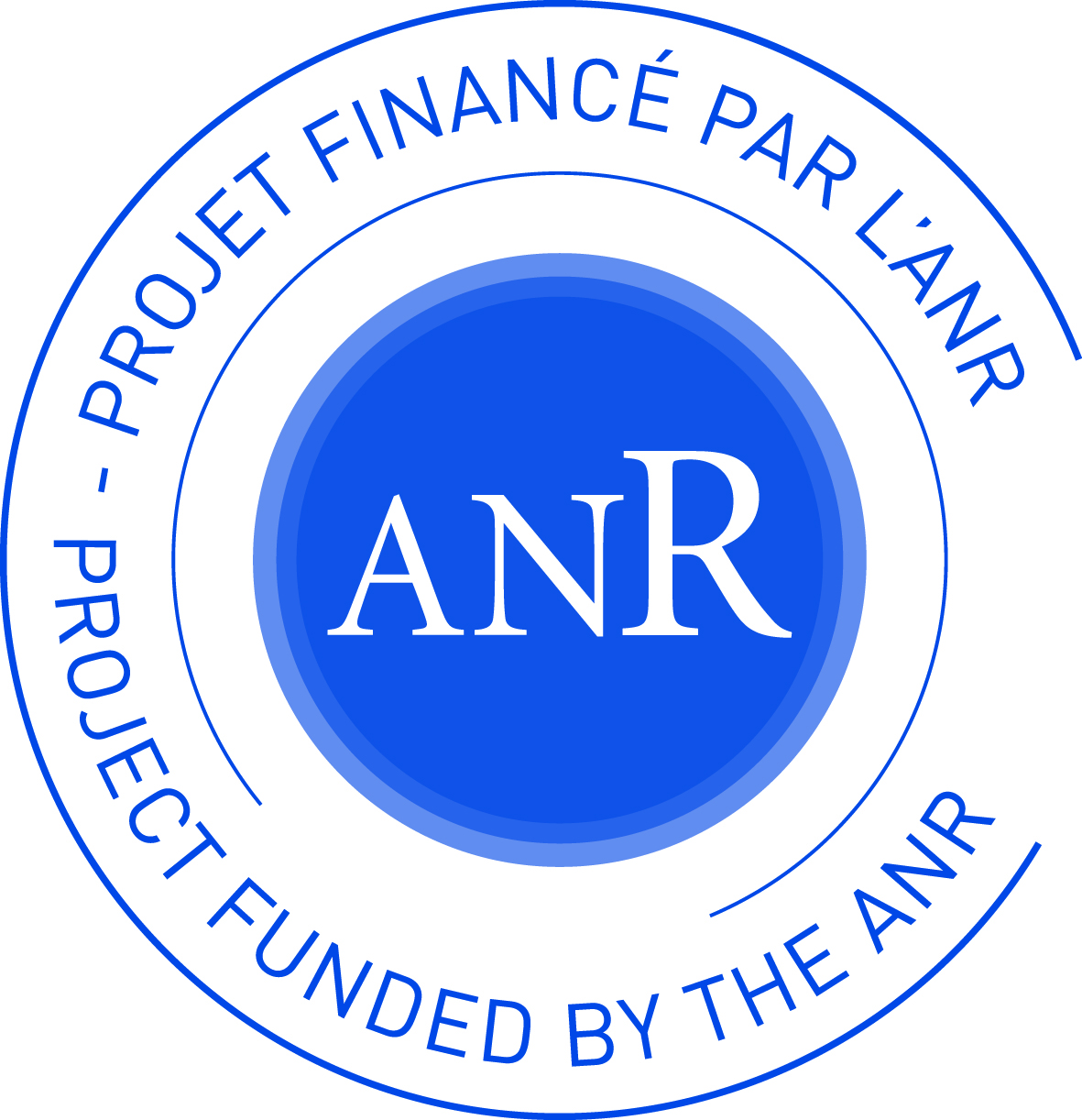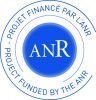## ANR-LSDLarge Stochastic Dynamics

agence-nationale-recherche.fr/Project-ANR-15-CE40-0020

Team

Publications ANR LSD

# Défi de tous les savoirs (DS10) 2015 Projet LSD

### Large Stochastic Dynamical Models in Non-Equilibrium Statistical Physics

Statistical mechanics provides the framework for relating macroscopic phenomena, both equilibrium ones like boiling and freezing, and nonequilibrium ones like heat conduction, diffusion, etc., to their origins in the dynamics of atoms and molecules. These fundamental issues, in particular in non-equilibrium, are not fully understood due to the lack of reliable numerical results and of sound analytic theories. This calls for mathematical analysis in order to provide rigorous results in specific models that could then settle some controversies in the physical theories and serve as benchmark.

In this mathematical project, we aim to use large stochastic dynamics in order to understand the non-equilibrium problems of statistical mechanics. We intend to use tools from probability theory as large deviations, non-linear stochastic PDE and systems with a large number of degrees of freedom. Solving these problems from statistical physics requires developping a better understanding of the mixing properties of Markov chains and diffusion processes.

In particular we will focus on the following issues:

• Macroscopic Transport: We want to understand how the macroscopic evolu- tion equations, like Euler equation or heat equation, arise from microscopic dynamics after a coarse graining and a proper rescaling of space and time. We will work on the properties of the stationary non-equilibrium states when the systems are driven out of equilibrium by the boundary condi- tions. We plan to study the corresponding transport coefficients, like the thermal conductivity, and the superdiffusion arising when these coefficients diverge in some low dimensional systems. We will also consider the fluc- tuations around the limit macroscopic behavior and in particular the large deviations.

• Slow and disordered dynamics: In some physical systems, like glasses, the dynamics are so slow that these systems never reach equilibrium within observable time scales. These dynamics play a key role in non-equilibrium physics and we are going to study three types of models where dynamical slowdown has very different origins: geometric constraints, condensation and disorder.

• Interface dynamics and KPZ equation: Interface dynamics are ubiquitous in non-equilibrium statistical mechanics, in modeling the evolution of phase boundaries, growth models, etc. According to the scale one is interested in, they can be effectively described via deterministic PDEs of mean-curvature type or by (highly singular) stochastic PDEs. Both regimes pose challeng- ing mathematical questions. Growth models are intimately related to the asymmetric lattice gases out of equilibrium.

### Partners

CMAP Centre de Mathématiques Appliquées de l'Ecole polytechnique

ICJ-CNRS Institute Camille Jordan - Centre National de la recherche Scientifique